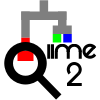# why some of the weighted unifrac distances are lager than one

Hi. After filtering ASV by certain criteria, I calculated weighted unifrac distance by “qiime diversity core-metrics-phylogenetic”. Somebody know why there are so many values lager than one? The biggest is 1.34657630162225. All the values in unweighted unifrac distance matrix are smaller than one.
Here is the code.
qiime taxa filter-table
–i-taxonomy taxonomy.qza
–p-include D_1__
–p-exclude Archaea,Eukaryota
#filter three samples of low sequencing depth
qiime feature-table filter-samples
#diversity caluculation
qiime diversity core-metrics-phylogenetic
–p-sampling-depth 10000

1 Like

Hi @yuqing,

Welcome to theforum!

It has to do with the way weight is calculated. In unweighted UniFrac, we calculate distance as \frac{\cap \textrm{ branches}}{\cup \textrm{ branches}} (Shared history / total history). So, this value is always between 0 and 1.

Weighted UniFrac is the sum of the the branch length by the absolute difference in count fractions \sum_{i}^{n}{b_{i} \mid \frac{A_{i}}{A_{T}} - \frac {B_{i}}{B_{T}} \mid } where b_{i} is the branch length, A_{i} is the total number of sequences in sample A along the length of the branch, A_{T} is the total number of sequences in sample A, B_{i} are the sequences in B along the branch and B_{T} the total sequences in B. But, because of this scaling, the distance can easily exceed one. There's also a rescaling factor that can be used to adjust for the fact that a longer branch length may correlate to more difference... I think it's probably easier for you to read the original paper than for me to try and dance around the math.

I do want to note that there's no requirements distances be between 0 and 1, though. I'm about to go to work, which is a distance of about 8 metro stops. I could theoretically rescale that as the fraction of the subway if that was somehow more meaningful here, but I can also just represent it as 8 subway stops. The issue is the relative scale, not the quantity. The one thing I can't do is compare my distance of 8 subway stops with someone else who's scaled their metro distance, because then it's not the same thing!

Best,
Justine

4 Likes

This topic was automatically closed 31 days after the last reply. New replies are no longer allowed.